# How to Find Vertical Asymptotes

## Asymptote, Vertical Asymptote

An asymptote is a line or curve that become arbitrarily close to a given curve. In another words it is a line close to a given curve, such that the distance between the curve and the line approaches zero when the curve is reaching higher/ lower values. The region of the curve that has an asymptote is asymptotic. Asymptotes are often found in rotational functions, exponential function and logarithmic functions. Asymptote parallel to the y-axis is known as a vertical asymptote.

## Determining the Vertical Asymptote

If a function f(x) has asymptote(s), then the function satisfies the following condition at some finite value C.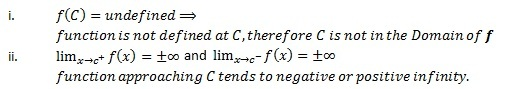In general, if a function is not defined at a finite value, it has an asymptote. Nevertheless, a function that is not defined at a point might not have an asymptote at that value if the function is defined in a special way. Therefore, it is confirmed by taking the limits at the finite values. If the limits at the finite values (C) tend to infinity, the function has an asymptote at C with the equation x=C.

## How to find vertical asymptotes – Examples

• Consider f(x)=1/x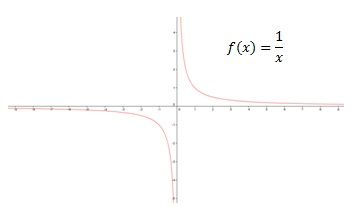Function f(x)=1/x has both vertical and horizontal asymptotes. f(x)is not defined at 0. Therefore, taking the limits at 0 will confirm.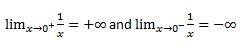Notice that the function approaching from different directions tends to different infinities. When approaching from negative direction the function tends to negative infinity, and approaching from positive direction the function tends to positive infinity. Therefore, the equation of the asymptote is x=0.

• Consider the function f(x)=1/(x-1)(x+2)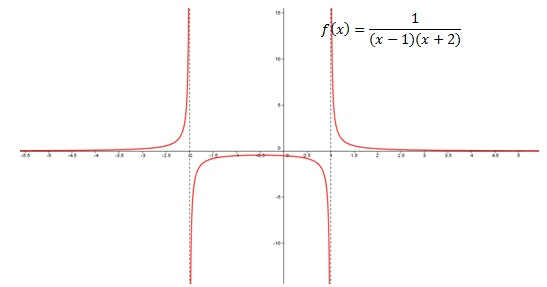The function does not exist at x=1 and x=-2. Therefore, taking limits at x=1 and x=-2 gives,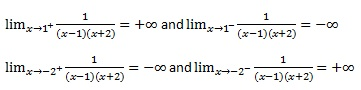Therefore, we can conclude that the function has vertical asymptotes at x=1and x=-2.

• Consider the function f(x)=3x2+ex/(x+1)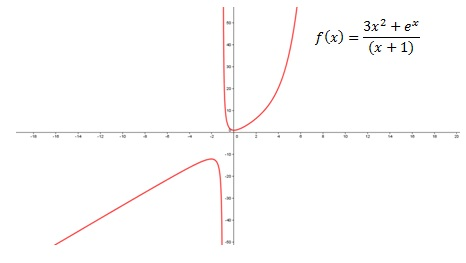This function has both vertical and oblique asymptotes, but the function does not exist at x=-1. Therefore, to verify the existence asymptote takes the limits at x=-1

Therefore, the equation of asymptote is x=-1.

A different method has to be employed to find the oblique asymptote.